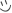# Linear Algebra Quiz

This linear algebra quiz tests your knowledge on some of the fundamental principles that are crucial in the field of linear algebra. A holisitic understanding in linear algebra is required to succeed on this quiz

The quiz covers many of the concepts taught in first year university courses of linear algebra. Take the quiz to get a sense of how well you understand linear algebra and what areas you need to improve on!

Created by: Yihao Xu

1. The determinant of any identity matrix is:
2. A matrix is not invertible if:
3. A system of m linear equations in n variables is inconsistent if:
4. A system of m linear equations in n variables cannot have a unique solution if:
5. A 4x4 matrix cannot be an elementary matrix if:
6. Mutiplying an nxn elementary matrix on the right of an mxn matrix A is equivalent to:
7. A set of k vectors in n dimension is a basis for R^n if:
8. An idempotent matrix:
9. The number of free variables in a system of m linear equations in n variables depends on:
10. Choose the statement that is true

You're about to get your result. Then try our new sharing options.